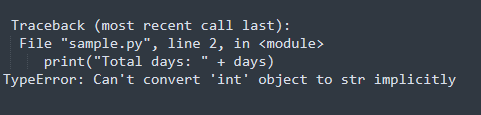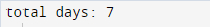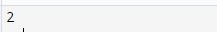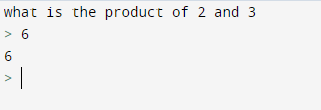# TypeError: Can’t convert int to str implicitly

Python generates the TypeError: can’t convert int to str implicitly when you try to join a string with an integer. Python does not allow the implicit concatenation of string and integer. However, you can do …Python generates the TypeError: can’t convert int to str implicitly when you try to join a string with an integer.

Python does not allow the implicit concatenation of string and integer. However, you can do so by using str(), a built-in function in Python, to concatenate these two data types explicitly.

## What is TypeError in Python:

TypeError is one of the exceptions in Python. TypeError generates when you try to operate on an incorrect object or data type.

For example, TypeError: can’t convert ‘int’ object to str implicitly.

### TypeError: can’t convert ‘int’ object to str implicitly

In programming, the data stores in many different ways. The data stores with respect to its data type. All the textual data will be stored in the data type string. The whole numbers will store in the data type integer and so on.

Python provides a lot of ease to the programmers. When working with python, especially beginners do a mistake a lot of time that they try to join two different data types implicitly with the help of a common concatenation method that is putting ‘+’ operator between the two objects.

The method of common concatenation is safe when you try to do string appending.

String appending is a method of joining or concatenating two strings with the help of the ‘+’ operator.

Beginners of python programming do a very common mistake in that they concatenate an integer with a string directly by using the ‘+’ operator.

Example:

``````days =7
print("Total days: " + days)
``````

Output:But as python is a very user-friendly programming language, it provides a lot of techniques to solve a problem and do the wanted task.

## Convert int to string – python:

As we know that string and integer cannot directly stick together. So, whenever you try to do this, it will give you an error.

Example:

``````days =7
print("Total days: " + days)
``````

Output:The TypeError generates because the ‘+’ sign does not work between integer and string. python considers the left operand “ Total days” as a string. And the right operand days as an integer. So, it gives an error because Python does not know how to add a string with an integer.

This problem can be resolve by explicitly parse the integer to a string by str() built-in function.

The str() function converts the integer to the string and thus you can easily concatenate them.

Syntax: string + str(integer)

Example:

``````days =7
print("total days: " + str(days))
``````

Output:However, there are other methods to solve this issue.

• print (“Total number of days: “, days)
• print (“Total number of days: {}”.format(days))

## Convert string to integer – Python:

In python, you can concatenate a string to an integer by converting string data type to integer data type. To do so use python’s built-in function int().

Syntax: int(number)

Example:

``````print(int("2"))
``````

Output:The int() function can pass two parameters. One is the string and other is the base that you want to give the converted string to integer. The base parameter is completely optional.

Syntax: int(number,base=base)

Example:

``````print(int("10" , base=3))
``````

Output:Now let’s convert the string to the integer with the help of above mentioned techniques.

Example:

``````product = input("what is the product of 2 and 3")
print(product)
``````

The example asks the user to enter an integer so that the string inside the variable product can be converted to int.

Output:### Conclusion:

Python is a very user-friendly programming language. It provides users a lot of easiness to code. However, it has some rules to follow.

The TypeError: can’t convert int object to str implicitly, is one of the common errors generate in the codes of the beginners.

The reason behind the generation of this error is that the programmer tries to concatenate string and integer directly by using the common ‘+’ operator.

The problem can solve the converting integer to string by using the str() function and string to an integer by using the int() function.

Suggested Read: 500 Internal server error